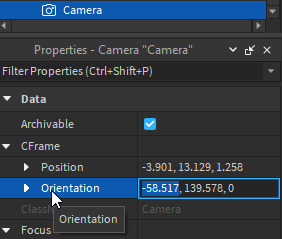# How to get the exact Orientation-Number of Camera?

print(Vector3.new(workspace.CurrentCamera.CFrame:ToEulerAnglesXYZ()))
But there is not the number coming out I want to see.

I need this number:Thanks!

What number are you getting and which do you want?
The orientation in the editor is in degrees, but fromEulerAngles will give it to you in radians.

Because it returns it in radians, you need to switch it to degrees.

``````local function radiansToDegrees(vector: Vector3): Vector3
return Vector3.new(
math.deg(vector.X),
math.deg(vector.Y),
math.deg(vector.Z)
)
end

local x, y, z = workspace.CurrentCamera.CFrame.Rotation:ToOrientation()
``````

The main unit for angle measurement in Roblox is Radians that’s why all angle functions return radians and it’s the same reason why you need to pass radians when calling an angle function (except some cases where it has been specified).

When printing Orientations of a CFrame, it will give the exact CFrame of the Orientation with Matrices, you need to convert them to XYZ using `ToOrientation`

``````local x,y,z = cf.Rotation:ToOrientation()
``````

What this will do is convert the CFrame Rotation to a XYZ format, which would return as radians, `math.deg()` would convert it to Degrees.

Radians are sort of simple to understand, but complicated for exact numbers.

`CFrame.Angles` (in fact CFrame in general) uses Radians as a Unit of Measurement, which uses the number `pi` for things,

180 degrees in radians is exactly `math.pi` and 90 would be `math.pi/2`, while the full rotation (360) is `math.pi*2`. To get exact numbers in radians would be very tedious to do it this way, so we use `math.rad` to help us with work, if you want to do it manually, It would be `x * (math.pi/180)`

So if you ever saw `math.pi/2` being used for something like this, thats why.

This topic was automatically closed 14 days after the last reply. New replies are no longer allowed.